# Adaptive filters: stable but divergent

## Abstract

The pros and cons of a quadratic error measure in the context of various applications have often been discussed. In this tutorial, we argue that it is not only a suboptimal but definitely the wrong choice when describing the stability behavior of adaptive filters. We take a walk through the past and recent history of adaptive filters and present 14 canonical forms of adaptive algorithms and even more variants thereof contrasting their mean-square with their l 2−stability conditions. In particular, in safety critical applications, the convergence in the mean-square sense turns out to provide wrong results, often not leading to stability at all. Only the robustness concept with its l 2−stability conditions ensures the absence of divergence.

## 1 Introduction: some historical background on adaptive-filter stability

The basic concept of a quadratic error measure whose minimum can simply be found by differentiating and solving a resulting set of linear equations, invented by C.F.Gauss in 1795, has been the tool of choice for about 200 years. In , many arguments were demonstrated to question the usefulness of the mean squared error (MSE) in image and audio processing due to our complex human perception and these arguments were nicely supported by many practical examples and observations.

Such a quadratic error measure has also been employed in adaptive-filter theory as a practical means to derive convergence in the mean-square sense, starting with Ungerböck in 1972  who applied the technique onto Widrow and Hoff’s famous least-mean-square (LMS) algorithm 1. He also introduced the so-called independence assumption that is not well argued for  but a necessity once MSE techniques are being applied. The concept was to evaluate not only the mean of the parameter-error vector $$\tilde {\mathbf {w}}_{k}={\mathbf {w}}-{\mathbf {w}}_{k}$$ (also known as weight error vector) but also the mean-square of it, typically in terms of the parameter-error vector covariance matrix $$E\left [\tilde {{\mathbf {w}}}_{k}\tilde {{\mathbf {w}}}_{k}^{\textsf {H}}\right ]$$. Although originally derived in the context of machine learning, the LMS algorithm is a standard gradient-type algorithm mostly applied for system identification (see Algorithm 1). Many other applications, such as linear prediction or active noise control , can be brought into such framework. Note that we refer to the estimates of the time-invariant reference w by w k , that is, time-variant estimates. This paper sticks with the estimation of time-invariant systems; to describe the tracking behavior of learning systems, another notation would be required. Figure 1 depicts a typical setup for system identification. For the LMS algorithm, simply set F=I. Note that we describe Algorithm 1 in terms of a finite impulse-response (FIR) filter. There are also other filter structures possible, e.g., infinite impulse-response (IIR) filters described in the next section or linear input combiners in which the input sequence u k is completely defined by instantaneous input vectors u k with typical applications in adaptive antenna beamforming .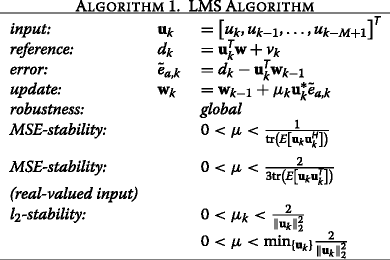Notation: Note that we use a short notation for the linear time-invariant operator $$F=F(q^{-1})=\sum _{i=0}^{M} f_{i} q^{-i}$$ with the unit-delay operator q −1 x k =x k−1. Analogously, $$F(e^{-j\Omega })=\sum _{i=0}^{M} f_{i} e^{-ji\Omega }$$ denotes the Fourier transform of the impulse response {f i }. F R denotes the corresponding backward operator, that is $$F^{R}=F^{R}(q^{-1})=q^{-(M+1)}\sum _{i=0}^{M} f_{M-i} q^{i}$$. For IIR filters, $$B=B(q^{-1})=\sum _{i=0}^{M} b_{i} q^{-i}$$ denotes the FIR part and $$A=A(q^{-1})=\sum _{i=1}^{M} a_{i} q^{-i}$$ the recursive part. Matrices are denoted in capital boldface letters; F>0 means that all eigenvalues of F are positive. Table 1 lists the most commonly used variable names and their meaning.

## 2 First stability problems found

Employing the MSE method as the favorite and satisfying tool of most researchers in the field of adaptive filters, Feintuch introduced in 1976 an adaptive algorithm  (see Algorithm 2) that exhibited first obstacles. He proposed the estimation of IIR filter coefficients (A,B), rather than the conventional FIR (B) coefficients, located in the filter weights w k . Usually, when a stability issue in adaptive filters occurs, practitioners recommend to lower the step-size μ k , thus buying increased stability with the expense of slower convergence. However, this method did not work for Feintuch’s algorithm.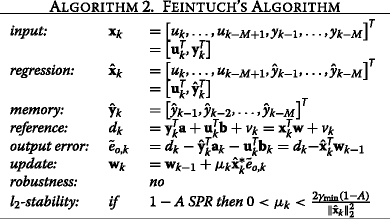Immediate responses [8, 9] after this publication showed that the MSE argumentation must be wrong even though the originating author with help by others valiantly defended his MSE-based argument. Soon after the notion of strict positive real (SPR)  was introduced (see Table 2). A linear time-invariant filter F is called SPR if for all frequencies −πΩπ the transfer function Re {F(e jΩ)}>0. Derived in the context of so-called hyperstability, it now became obvious that adaptive IIR filters —no matter if updated with a gradient-type algorithm or a more complex Gauss-Newton-type algorithm—all share a common fate; the output error signal used for updating the filter weights passes first through a linear filter $$\tilde {e}_{o,k}=v_{k}+\frac 1{1-A}\left [\hat {\mathbf {x}}_{k}^{\textsf {T}}\tilde {\mathbf {w}}_{k-1}\right ]$$ (set filter F=1/[ 1−A] in Fig. 1). If this filter exhibits the SPR property, a step-size small enough can be found to ensure convergence of the algorithm, while if such property is not satisfied, sequences can be found for which the filter not only does not converge but indeed diverges for all step-sizes (that are nonzero). It now became obvious why some situations worked for this algorithm and others did not. Once the recursive part of the coefficients in 1−A does not satisfy the desired SPR condition, the algorithm is doomed to be unstable. The history of this development is nicely summarized in  and the interested reader is recommended to read it.

In fact, Feintuch’s adaptive IIR filter algorithm is a special case of a so-called filtered-error-type algorithm (see Algorithm 4). A very simple instantiation of such filtered-error-type of algorithm is the so-called LMS algorithm with delayed updates (DLMS) . It occurs if the error filter is a simple delay, which can easily happen if a pipelined chip structure for the LMS algorithm is designed that requires to introduce a delayed version of the error signal. If the error signal appears delayed by, say K>0 steps, the filter F(e jΩ)=e jKΩ cannot be SPR and thus a pipelined LMS algorithm can become unstable. However, the cure for this algorithm is simply obtained by also delaying the regression vector by K steps and applying an older estimate, as shown in Algorithm 3.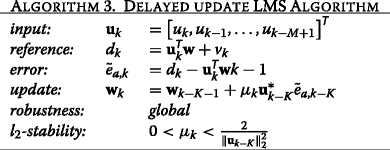If the filter F is known and non-SPR, a cure can be rather simple by applying an additional backward filter F R to the filtered error. This results in an SPR part F F=|F|2 and a pure delay e jMΩ that can be treated by delaying the regression vector u k in a similar way to the DLMS cure in Algorithm 3. Note, however, that such treatment usually results in a rather slow update rate as the error signal is severely delayed now.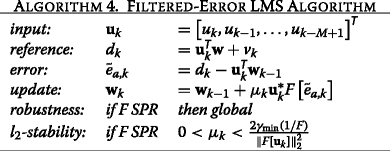Such a behavior was also detected in the context of active noise control, where the linear filter F is not defined by the unknown recursive part of an IIR filter but by an acoustic-electrical transfer function, defined also by the mechanical construction of the concatenation of loudspeaker, free-space, and microphone system . Different from the adaptive IIR filter, however, in acoustic noise control, the filter F can be observed and its impulse response identified first and then compensated for. An alternative idea that avoids applying the backward filter was proposed by applying the error filter F on the regression vector. Many algorithms being derivatives of this so-called Filtered-X-LMS algorithm (see Algorithm 5) have been proposed during the 1980s to overcome the SPR condition, once F is known. The essential idea is to compensate the impact of the filtered error by an identical filter on the regression vector (in this case G k [.]=1). In , robustness conditions for the Filtered-X-LMS algorithm were analyzed and it was found that, although placing F on the regression vector has a beneficial behavior, the algorithm is in general only locally robust. The Filtered-X-LMS algorithm was then reformulated in the form of a filtered-error-type; however, now, a new, time-variant linear operator 1/[1−μ k C k ] applies on the filtered error. The coefficients of this time-variant operator C k depend on linearly filtered versions of the input signal u k as well as on the algorithm’s step-size. As the coefficients of μ k C k are proportional to the step-size μ k , sufficiently small step-sizes can ensure that 1−μ k C k is SPR, however, with the price of a slowed down adaptation. Only if a particular time-variant linear operator $$G_{k}=G_{o}=\frac 1{1+\mu _{k} C_{k}}$$ is additionally applied on the filtered error term, this can be compensated for and the algorithm can be sped up considerably.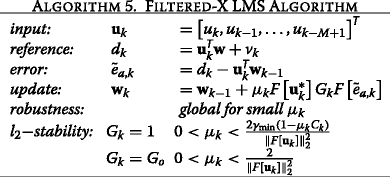The Filtered-X LMS algorithm has experienced a renaissance during the past years as it appears to be the right choice for vibration control in car engines . A novel aspect here is that car engines can be controlled without sensors as the engine speed is known. The input signal u k can thus be generated artificially out of weighted sine and cosine terms of the car’s rotation frequency Ω and multiples thereof. A compact notation of this algorithm results in a complex-valued Filtered-X LMS algorithm. However, due to physical reasons, the error signal must be real-valued and therefore a complex-valued LMS algorithm is run only by a real-valued error fraction. In , it was shown that this variant behaves indeed in a robust way while an alternative variant employing a complex-valued error and a real-valued regressor does not. Both variants show identical MSE behavior though.

## 3 Stability of adaptive filters

After so much disturbing news on potential instability, it is time now to take a closer look into the stability of adaptive filters as we need to understand the various notions of stability.

MSE-stability: is based on minimizing $$E[|\tilde {e}_{a}|^{2}]$$ with respect to the parameter estimates w k [2, 2226]. Depending on the application, a minimal remaining error energy can be desired (signal adaptation), but also the correct knowledge of the parameters w may be desired (system adaptation). In the classical MSE analysis, the parameter vector error-covariance matrix $$\mathbf {P}_{k}=E\left [\tilde {\mathbf {w}}_{k}{\tilde {\mathbf {w}}}_{k}^{\textsf {H}}\right ]$$ is studied—requiring the so-called independence assumptions on the participating processes u k and v k —and step-size conditions are derived to guarantee tr(P k ) to decrease. Due to this procedure, MSE-stability always includes some form of convergence. If an additive stationary noise process v k is assumed, the algorithm converges into a nonzero steady-state.

l 2stability: is based on robustness terms originating from control theory [27, 28] in the form of l 2-norms of instantaneous regression vectors rather than their expectation values. In the context of adaptive filters, it was introduced in 1993 by Kailath, Sayed, and Hassibi . Further work over the next 10 years [25, 3033] showed that more and more adaptive filters exhibit such property. In loose words, l 2−stability simply says that if the input sequence has a bounded Euclidean norm, so does the output sequence. Note that, different from common treatment, inputs of the scheme are now additive noise v k as well as the initial parameter-error vector $$\tilde {\mathbf {w}}_{0}=\mathbf {w}-\mathbf {w}_{0}$$, outputs are the undistorted a-priori error sequence $$e_{a,k}=\mathbf {u}_{k}^{\textsf {T}} \tilde {\mathbf {w}}_{k-1}$$ and possibly the a-posteriori parameter-error vector $$\tilde {\mathbf {w}}_{k}=\mathbf {w}-\mathbf {w}_{k}$$. The driving sequence u k only influences the algorithmic mapping from input to output.

Different from MSE-stability, the robustness concept leading to l 2−stability does not require any simplifying assumptions on the signals and formulates the adaptive learning process in terms of a feedback filter structure with an allpass (unitary transformation) that is lossless in the feedforward path and a feedback path that usually contains all important signal and system properties as well as free parameters such as the step-size. A typical structure of this for the standard LMS algorithm with time-variant step-size μ k is shown in Fig. 2.

As the stability result depends on the small-gain theorem [27, 28], the resulting step-size bound is conservative. While for the classic LMS algorithm, the observation coincides very sharply with the predicted bounds, for many other algorithms, the bound obtained is indeed conservative.

In the context of robustness, the question about worst-case sequences was posed the first time: what sequences {w 0,v 0,v 1,…,v N } can be envisaged for the worst behavior of the LMS algorithm, i.e.,

$$\max_{\{\mathbf{w}_{0},v_{0},v_{1},\ldots,v_{N}\}} \frac{\|\tilde{\mathbf{w}}_{N}\|_{2}^{2}+\sum_{k=1}^{N} \mu_{k} |e_{a,k}|^{2}}{\|\tilde{\mathbf{w}}_{0}\|_{2}^{2}+\sum_{k=1}^{N} \mu_{k} |v_{k}|^{2}}\le \gamma ?$$
((1))

For gradient-type algorithms it was concluded that, if the noise sequence compensates the undistorted error, i.e., v k =−e a,k , the algorithms do not update and their maximum robustness level γ is obtained with identity, thus such sequences were considered to cause worst-case situations. Surprisingly, there was no worst condition imposed on the driving sequence u k as long as $$0<\mu _{k}<2\bar {\mu }_{k}=\frac 2{\|{\mathrm {u}_{k}}\|_{2}^{2}}$$. The reason for this may be in the fact that the method itself aims for a convergence of the undisturbed error sequence $$e_{a,k}={{\mathbf {u}_{k}^{\textsf {T}}}} \tilde {{\mathbf {w}}}_{k-1}\rightarrow 0$$, rather than the parameter-error vector $$\tilde {{\mathbf {w}}}_{k}$$. Not surprisingly, signal conditions only came up when requiring that not only the error energy of |e a,k |2 tends to zero but also that the parameter-error vector $$\tilde {{\mathbf {w}}}_{k-1}={\mathbf {w}}-\hat {{\mathbf {w}}}_{k-1}$$, i.e., the difference between the true system impulse response and its estimate, converges strongly (in norm) to zero. If the latter is also required, the driving signal vectors u k need to be persistent exciting, i.e., consecutive vectors need to span the space of dimension M, if M denotes the filter order.

A consequence of the l 2−stability property is that an energy-bounded input sequence (noise v k and initial parameter-error vector $$\tilde {{\mathbf {w}}}_{0}$$) causes a bounded output of undistorted errors e a,k . If the input sequence is a Cauchy sequence, so is the output. If, on the other hand, such bound γ cannot be guaranteed, it is likely that an input sequence exists that causes divergence. Convergence in this context means that a range of step-size parameters (or alternative design parameters) exist, for which even under worst-case sequences, no divergence occurs.

The concept of l 2−stability is thus very different from MSE-stability as the existence of a single worst-case sequence (e.g., one among an infinite amount) still would guarantee MSE-stability (an infinite amount of working sequences outweigh the single worst-case sequence) but not vice versa. The idea of l 2−stability is thus more restricting and to be preferred in cases where security is of utmost importance (smart cities, smart grids, transportation flow, automatically controlled cars, flight control, and so on), while MSE-stability might only be sufficient for typical applications in telecommunications where corrupted data transmissions can be corrected by different means. We can conclude that for bounded random sequences, l 2-stability leads to MSE-stability but not to the opposite.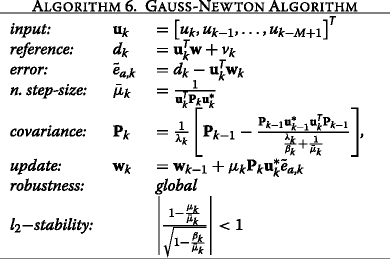The robustness framework was even able to handle such different algorithms as the Gauss-Newton-type Algorithm 6, of which the recursive least squares (RLS) algorithm is its most famous special form, but also single-layer neural network adaptations. The global robustness and l 2−stability of the RLS algorithm was shown , corresponding results for the entire Gauss-Newton algorithmic family with time-variant forgetting factor 0<λ k <1 as well as memory factor 0<β k ≤1 are reported in , special results for least squares (LS) estimators including Kalman filters appeared in . The real-valued Perceptron learning algorithm (PLA), see Algorithm 7, was shown to be l 2−stable in . Even more complicated single-layer structures, such as the so-called Narendra and Parthasarathy structure, that include feedback with memory could be analyzed and l 2−stable conditions were provided.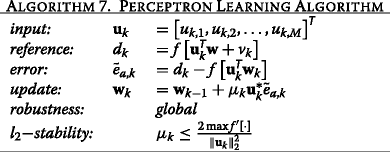## 4 Recently discovered evidence

Until here, the occurrence of a linear filter in the error path may have been regarded as some curiosity in the many variations of adaptive-filter algorithms and applications that simply set an exemplary exception, requiring a different treatment while the majority of adaptive-filter algorithms work accurately according to an MSE-based theory. The developed robustness description allows to define stability conditions for all those cases very accurately.

Back to our historical walk. In the 1990s, adaptive filters for neural networks and particular fast versions of LS techniques were in the focus, so called Fast-RLS algorithms. Their theory is also based on minimum MSE (MMSE) but, due to their deterministic nature, independence assumptions were not required. To include them in practical applications, their LS nature was often sacrificed, and time-variant step-sizes were introduced. With such step-sizes, however, their nature was more along the stochastic, gradient-type algorithms. One of these RLS derivatives is the affine projection (AP) algorithm  that speeds up convergence when compared to its simpler gradient counterpart by taking P past regression directions into account. A fast version of this [39, 40] is the basis for millions of copies of such algorithms running today in electric echo cancellation devices to reduce the echoes of long-distance call telephone cables. Unlike the original algorithm, they use a sophisticated step-size control to prevent instable behavior in double-talk situations , that is when both talkers are active. The resulting algorithm is called pseudo affine projection (PAP) algorithm, see Algorithm 8, as with a moderate step-size the original property is lost. Recently, it has been shown  that depending on the correlation of the input signal, such PAP algorithm can become unstable and that, depending on the input signal statistic, situations exist in which even small step-sizes do not result in stable behavior but larger ones are required; thus depending on the steady-state of the predictor coefficients a k (correspondingly denoted here as linear operator A(q −1)), lower normalized step-sizes α min may exist as well as upper bounds α max.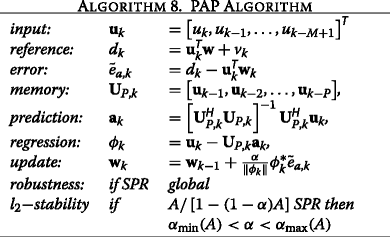However, this is not the only algorithm for which stability problems remained undiscovered for a long time. A well-known adaptive algorithm for zero forcing (ZF) equalization is the gradient algorithm by Lucky , see Algorithm 9. In the well-known text book by Proakis  we can read:

“The peak distortion has been shown by Lucky (1965) to be a convex function of the coefficients. That is, it possesses a global minimum and no relative minima. Its minimization can be carried out numerically, using, for example, the method of steepest descent”.

The argumentation sounded very convincing until the algorithmic behavior was analyzed throughly in  and it was found that indeed there exist channel conditions and data sequences that cause the algorithm to diverge, even for smallest step-sizes. Based on the channel impulse response {h i }, step-size conditions only for MSE-stability can be derived. See also  for alternative non-robust ZF equalizer algorithms.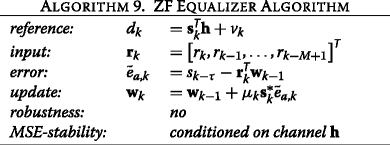Such examples may corroborate the suspicion that they all may be related to a linear filter in the error path of some form and thus depend on an SPR condition. Note, however, that neither for the ZF algorithm nor for the PAP algorithm, any SPR condition appears in the error path; thus, they do not fall under the existing knowledge of the early 1990s, their l 2− stability behavior being much different from their MSE behavior. In the meantime, they were, however, correctly analyzed by the now existing robustness techniques [42, 45].

Moreover, also other problems can cause stability trouble, when the driving signal is of persistent excitation. Once we consider algorithms with matrix inverses such as RLS algorithms, it is well understood that with a lack of persistent excitation, a null space in the solution opens up that offers the algorithm a wide space to diverge. Also in applications, such as stereo hands-free telephones , null-spaces can occur as part of the solution and cause adaptive filters to diverge. In such cases, regularization and leakage factors are often applied to force the null spaces out of the obtained estimates.

But the existence of null-spaces is not necessarily a reason for a lack of robustness. It may thus come as a surprise that there exists an adaptive algorithm for blind channel estimation [48, 49] that is indeed robust [46, 50], although it is known that most of the blind methods are non-robust ; see Algorithm 10 for details. It is the classical two-channel path setup as depicted in Fig. 3, in which the task of the algorithm is to estimate both channels, g and h, denoted here as concatenated vector $${\mathbf {w}}_{k}={\left [{{\mathbf {g}}_{k}^{\textsf {T}}},{{\mathbf {h}}_{k}^{\textsf {T}}}\right ]^{\textsf {T}}}$$. This result, however, does not mean that all blind algorithms are robust; some more comments on this topic are provided in Section 8.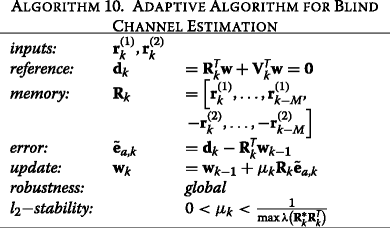## 5 A converse approach: worst-case scenarios that lead to divergence

While for many known adaptive filters, it was now possible to show robustness conditions; for some of them, the problem remained unsolved as they cannot be brought into the feedback structure as depicted in Fig. 2. Typically, these problematic adaptive filters employ the general update form:

$${\mathbf{w}}_{k}={\mathbf{w}}_{k-1}+\mu_{k}{\mathbf{x}^{\ast}_{k}}\tilde{e}_{a,k},$$
((2))

that is directions x k are applied, different (not parallel) from driving process vector u k that constitutes the error $$\tilde {e}_{a,k}={e}_{a,k}+v_{k}={{\mathbf {u}^{\textsf {T}}_{k}}}\tilde {{\mathbf {w}}}_{k-1}+v_{k}$$. We refer to these algorithms in the following as asymmetric in contrast to symmetric algorithms, such as LMS or RLS. Equivalently speaking, it remained unclear for those adaptive filters of general asymmetric structure whether worst-case sequences exist that could cause divergence no matter what the step-size (μ k >0) is.

A more general view that encompasses also the driving processes u k into the worst-case scenarios and aims directly at the convergence or divergence of the parameter-error vector $$\tilde {{\mathbf {w}}}_{k}$$ is proposed in  where a similar argument to robustness is employed but instead of using the small-gain theorem, the sub-multiplicative property of norms in the context of SVD is applied.

The idea is the following: assume for simplicity the noiseless case and consider the parameter-error vector $$\tilde {{\mathbf {w}}}_{k-1}$$. An arbitrary gradient method may use its update error $$e_{a,k}={{\mathbf {u}_{k}^{\textsf {T}}}}\tilde {{\mathbf {w}}}_{k-1}$$ and update the parameter-error vector through

$$\tilde{{\mathbf{w}}}_{k}=\mathbf{B}_{k}\tilde{{\mathbf{w}}}_{k-1}=\left[{\mathbf{I}}-\mu_{k}{\mathbf{x}^{\ast}_{k}}{{\mathbf{u}_{k}^{\textsf{T}}}}\right]\tilde{{\mathbf{w}}}_{k-1},$$
((3))

that is, not necessarily into direction u k but x k . Applying the update several times results in a product of matrices $$\prod \mathbf {B}_{k}$$ whose largest singular value should remain bounded to preserve stability. This is equivalent to requiring a norm on B k to remain bounded and as $$\|\prod \mathbf {B}_{k}\|\le \prod \| \mathbf {B}_{k}\|$$ for many norms (sub-multiplicative property), we can conclude that l 2−stability can be guaranteed as long as the largest singular value σ max(B k )≤1. This condition is—similar to the small-gain theorem that was applied before—a conservative condition. However, due to the linear operators involved, it is now simpler to analyze converse conditions, i.e., bounds for instability rather than stability. Note that for the above example, the largest singular value turns out to be larger than one if x k α u k , that is if these vectors are not parallel.

If observation noise is added again, compared to (1), now, conditions of the form

$$\max_{\{{\mathbf{w}}_{0},v_{0},v_{1},\ldots,v_{N}\}} \frac{\|\tilde{{\mathbf{w}}}_{N}\|_{2}^{2}}{\|\tilde{{\mathbf{w}}}_{0}\|_{2}^{2}+\sum_{k=1}^{N} \tilde{\mu}_{k} |v_{k}|^{2}}\le \tilde{\gamma}$$
((4))

are obtained, which are obviously weaker than the original ones as the terms of the undistorted errors $$\sum _{k=1}^{N} \mu _{k} |e_{a,k}|^{2}$$ are missing. Similarly, to the robustness method of the previous section, stability conditions for the step-size μ k and boundedness conditions on additive noise can be derived now. However, the so obtained bounds appear to be tighter (or equivalent) when compared to the previous ones based on robustness. If the largest singular value of the mapping B k is larger than one, $$\tilde {\gamma }$$ will not remain bounded for N, growing to infinity, and thus robustness is potentially lost.

A good first example is the LMS algorithm, i.e, Algorithm 1. The classic robustness scheme showed l 2−stability as long as $$\mu _{k}<2/\|{\mathbf {u}_{k}}\|_{2}^{2}$$. But, how much larger can μ k become until the algorithm really diverges? Due to the conservatism of the small-gain theorem, we cannot answer this. The SVD method, on the other hand, allows to derive the worst-case sequence u k so that divergence can be guaranteed  and indeed for $$\mu _{k}>2/\|{\mathbf {u}_{k}}\|_{2}^{2}$$ divergence can be ensured that is, sequences that cause divergence can always be found. The stability bound of the LMS algorithm is thus tight as both methods deliver the same bound. While the SVD-based method provides an identical bound in this case; in many other algorithms, larger bounds could be identified.

Note, however, that the condition of having a singular value larger than one and thus the loss of robustness does not necessarily mean that the system must behave in an unstable manner. In order to cause instability, the driving signal must ensure that with every update step (or the majority of update steps), the condition is violated and not just once. As sometimes additional constraints are imposed to the adaptive filter, this potential worst-case sequence may not exist, and the algorithm may behave in a robust manner although one singular value is larger than one. Limitations of worst-case sequences typically occur with additional constraints due to the filter application and structure. A linear combiner with input u k from an arbitrary alphabet is very likely to cause a worst-case sequence leading to divergence, while an adaptive filter of FIR structure allows only one degree of freedom per iteration, as all other elements of the update vectors are already given. If the driving sequence is further restricted by originating from a limited alphabet, say binary phase shift keying (BPSK), it can very well happen that a singular value larger than one exists, but the excitation can never work in direction of its corresponding vector. A systematic example is provided further ahead in the context of the PNLMS algorithm. This brings us to the question of systematically finding worst-case sequences. For the above mentioned example, this is equivalent to requiring

$$\max_{{\mathbf{x}_{k}},{\mathbf{u}_{k}}} \sigma_{\max}\left({\mathbf{I}}-\mu_{k}{\mathbf{x}^{\ast}_{k}}{{\mathbf{u}^{\textsf{T}}_{k}}}\right) {\le 1}$$

for some positive range of step-sizes, for example 0<μ k <x k ,u k . Indeed for arbitrary vectors x k ,u k , it is relatively straightforward to find such sequences and prove divergence. However, as soon as x k =f(u k ) and the application imposes more restrictions, finding the sequences can become challenging.

It is very illustrative to view in this context the so-called proportionate normalized LMS (PNLMS) algorithm as a second example. Originally derived by Duttweiler  in 2000, the algorithm can be viewed as a time-variant counterpart of the algorithm by Makino ; both variants are shown in Algorithm 11. During the next 10 years the algorithm became very popular as a clever control of the diagonal step-size matrix can cause a significant speed up of the algorithm . Note that time-invariant matrix step-sizes that are positive definite or exhibit SPR properties are shown to be robust in [32, 42], ensuring l 2−stability of Makino’s algorithm. This can easily be shown as the product of consecutive matrices B k is equivalent to Eq. (5).

$$\begin{array}{@{}rcl@{}} \left({\mathbf{I}}-\mu_{k}\mathbf{L}{\mathbf{u}^{\ast}_{k}}{{\mathbf{u}^{\textsf{T}}_{k}}}\right)&\!\left({\mathbf{I}}-\mu_{k-1}{\mathbf{L}\mathbf{u}^{\ast}_{k-1}}{\mathbf{u}^{\textsf{T}}_{k-1}}\right) \\&\ldots \left({\mathbf{I}}-\mu_{1}{\mathbf{L}\mathbf{u}^{\ast}_{1}}{\mathbf{u}^{\textsf{T}}_{1}}\right){\tilde{\mathbf{w}}}_{0} \\ =\mathbf{L}^{\frac12} \left(\!{\mathbf{I}}-\mu_{k}\mathbf{L}^{\frac12}{\mathbf{u}^{\ast}_{k}}{\mathbf{u}^{\textsf{T}}_{k}}\mathbf{L}^{\frac12}\!\right)&\! \left(\!{\mathbf{I}}-\mu_{k-1}\mathbf{L}^{\frac12}{\mathbf{u}^{\ast}_{k-1}}{\mathbf{u}^{\textsf{T}}_{k-1}}\mathbf{L}^{\frac12}\!\right) \\ &\ldots \left({\mathbf{I}}-\mu_{1}\mathbf{L}^{\frac12}{\mathbf{u}^{\ast}_{1}}{\mathbf{u}^{\textsf{T}}_{1}}\mathbf{L}^{\frac12}\right) \mathbf{L}^{-\frac12}{\tilde{\mathbf{w}}}_{0}. \end{array}$$
((5))

The asymmetric form of matrix B k can thus be made symmetric and standard theory can be applied. Duttweiler replaced L by a time-variant diagonal matrix L k for which such symmetry correction in the style of (5) does not work any more. He showed his algorithm to be mean-square convergent. First attempts for showing robustness, however, turned out to require further rather limiting conditions on L k . In , it finally is shown that the PNLMS algorithm can indeed become non-robust even if the positive definite entries of L k are fluctuating only little.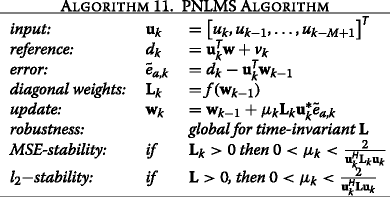## 6 Linearly-coupled and partitioned adaptive filters

We are further interested in understanding the stability of adaptive filters with asymmetric update forms. To this end, we apply the SVD-based method to so-called linearly-coupled adaptive filters where two adaptive filters use linear combinations of their error terms, that is

$$\left[\begin{array}{c} \mu_{g,k} \tilde{e}_{g,k}\\ \\ \mu_{h,k} \tilde{e}_{h,k}\end{array}\right] =\left[\begin{array}{cc} \nu_{1,k}&\nu_{2,k}\\ \\ \nu_{3,k}&\nu_{4,k}\end{array}\right] \left[\begin{array}{c} d_{g,k}-{\mathbf{u}^{\textsf{T}}_{k}}{\mathbf{g}}_{k-1} \\ \\ d_{h,k}-{\mathbf{x}^{\textsf{T}}_{k}}{\mathbf{h}}_{k-1} \end{array}\right].$$
((6))

Such a coupling may be undesired and caused by implementation or desired to achieve particular convergence properties . In case of two coupled adaptive filters, the four coupling factors ν 1,k ,ν 2,k ,ν 3,k ,ν 4,k can also consume the step-sizes so that only four degrees of freedom remain. Figure 4 depicts the setup. This structure turns out to be the vehicle to analyze cascaded and partitioned adaptive algorithms and is thus of high interest.

In a partitioned algorithm, the input vector is split up into one or more sections that run with a different (individual) step-size. This can facilitate parallel implementation and/or improved convergence speed. To simplify matters, let us thus envisage a simple form of a gradient algorithm in which we use two partitions with different step-sizes μ g,k and μ h,k . We split the entire parameter-error vector into two parts, say g and h, and correspondingly we use two partitions, say u k and x k , as regression vectors. The so obtained Bipartite PNLMS algorithm is summarized in Algorithm 12.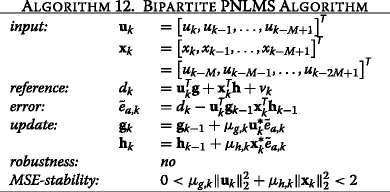Based on such an algorithmic formulation, we recognize that the bipartite PNLMS algorithm is of the same kind as linearly-coupled adaptive filters with the special step-size/coupling factor choice: ν 1,k =ν 2,k =μ g,k and ν 3,k =ν 4,k =μ h,k . Even if the two step-sizes are not identical, the update error is still linearly dependent for both partitions, causing one singular value to be larger than one, thus violating robustness. Only the weaker MSE-stability remains. In the following, we demonstrate this behavior on a simple example in which we first run the PNLMS algorithm with worst-case sequences rather than random sequences.

Bipartite PNLMS simulation example: we simulate the Bipartite PNLMS algorithm for a filter of length M=10 and average over 20 Monte Carlo (MC) runs. We vary the filter structure {linear combiner, FIR} as well as the input symbols {Gaussian, bipolar}. We choose a diagonal matrix

$$\begin{array}{@{}rcl@{}} \mu_{k}\mathbf{L}_{k}&=&\mu_{k} \left[\begin{array}{cc}1&0\\0& \rho_{k}\end{array}\right] {\otimes} \mathbf{I}=\mu_{k}\left[\begin{array}{cc}\mathbf{I}&0\\0& \rho_{k}\mathbf{I}\end{array}\right] \\ &=&\left[\begin{array}{cc}\mu_{g,k}\mathbf{I}&0\\0& \mu_{h,k}\mathbf{I}\end{array}\right] \end{array}$$
((7))

which nicely reveals the chimera character of the algorithm, being of PNLMS-type with a time-variant diagonal matrix L k as well as being of partitioned structure. If we further add the error terms, we recognize that

$$\begin{array}{@{}rcl@{}} \mu_{k}\mathbf{L}_{k}\tilde{e}_{a,k}&\!\!=&\!\!\left[\begin{array}{cc}\mu_{g,k}\mathbf{I}&0\\0& \mu_{h,k}\mathbf{I}\end{array}\right]\left[d_{k}-{\mathbf{u}^{\textsf{T}}_{k}}{\mathbf{g}}_{k-1}-{\mathbf{x}^{\textsf{T}}_{k}}{\mathbf{h}}_{k-1}\right] \\ &\!\!=&\!\!\left[\begin{array}{cc}\mu_{g,k}\mathbf{I}&\mu_{g,k}\mathbf{I}\\ \mu_{h,k}\mathbf{I}& \mu_{h,k}\mathbf{I}\end{array}\right] \left[\begin{array}{c} d_{k}/2-{\mathbf{u}^{\textsf{T}}_{k}}{\mathbf{g}}_{k-1}\\ d_{k}/2-{\mathbf{x}^{\textsf{T}}_{k}}{\mathbf{h}}_{k-1}\end{array}\right] \end{array}$$
((8))

which also reveals the linearly-coupled nature of the algorithm. We apply a small normalized step-size of $$\mu _{k}=0.1/\left [\|{\mathbf {x}_{k}}\|_{2}^{2}+\rho _{k}\|{\mathbf {u}_{k}}\|_{2}^{2}\right ]$$ for which all analyzed cases exhibit MSE-stability. In the first case, we set ρ k =ρ=2 and refer this to the fixed matrix L in Fig. 5, while in the second case, we select ρ k to be a random process uniformly distributed between zero and two and refer this to the random matrix L k in Fig. 6. While for a fixed matrix, the system mismatch has some potential to grow initially, it cannot keep growing and runs into a steady-state. Note that for bipolar driving signals as well as FIR filter structures, the worst-case sequences are relatively simply found as only a finite space has to be exhaustively searched through. For a uniform input (in [ −10,10]) and a linear combiner structure, a more sophisticated search is required though. As we recognize in Fig. 6, employing FIR filters restricts the search space for worst-case sequences dramatically and the filter converges despite its largest singular value being larger than one. This is different from a general PNLMS algorithm with arbitrary L k where we observe that having FIR filter structures is usually not a sufficient constraint to ensure robustness. The PNLMS algorithm with its time-variant diagonal matrix L k is thus an excellent example for an adaptive algorithm that is MSE stable but can behave in a divergent manner.

Cascaded or concatenated structures of adaptive filter algorithms have attracted many researchers in the past. The motivation can be as simple as dividing a long filter in shorter autonomous parts or owing to structural purposes [59, 60]. In the context of the identification of non-linear power amplifiers of large bandwidth, a concatenation of linear filter parts with memory and nonlinear parts without memory is very common. Depending on whether the linear filter comes first or not, we distinguish so-called Wiener or Hammerstein models [61, 62].

We pick here the Wiener filter structure as an illustrative example. The architecture is depicted in Fig. 7. A linear filter g is followed by a nonlinear filter h. The coefficients in h are linear weights to nonlinearly mapped instantaneous outputs of g, which serve as the input of h. Typically polynomial families with orthonormal properties such as Hermite or Legendre polynomials are applied. Consider a polynomial base {p i (x)};i=1,2,…,M. The gradient-type procedure is provided as Algorithm 13. In , only local robustness is shown for these algorithms. In , the algorithm is identified as a bipartite form of the PNLMS algorithm, as addressed in the previous section. We thus conclude that cascaded algorithms in general are potentially non-robust algorithms.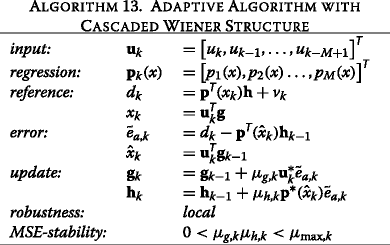Let us take a closer look at the uncorrupted update error based on the reference model p T(x k )h:

$$\begin{array}{@{}rcl@{}} e_{a,k}&=&{\mathbf{p}^{\textsf{T}}}(x_{k}){\mathbf{h}}-{\mathbf{p}^{\textsf{T}}}(\hat{x}_{k}) {\mathbf{h}}_{k-1}\\ &=&\left[{\mathbf{p}^{\textsf{T}}}(x_{k})-{\mathbf{p}^{\textsf{T}}}(\hat{x}_{k})\right]{\mathbf{h}} + {\mathbf{p}^{\textsf{T}}}(\hat{x}_{k}) \left[{\mathbf{h}}-{\mathbf{h}}_{k-1}\right] \\ &=& \left[x_{k}-\hat{x}_{k}\right] {\mathbf{d}^{\textsf{T}}_{k}} {\mathbf{h}} + {\mathbf{p}^{\textsf{T}}}(\hat{x}_{k}) \left[{\mathbf{h}}-{\mathbf{h}}_{k-1} \right]. \end{array}$$
((9))

We introduce a new vector $$\mathbf {d}_{k}=\left [p_{1}^{'}(x),p_{2}^{'}(x),\ldots,\right.$$ $$\left.p_{M}^{'}(x)\right ]^{\textsf {T}}$$ whose entries are simply the values of $$p_{i}^{'}(x)=\left [p_{i}(x_{k})-p_{i}(\hat {x}_{k})\right ]/\left [x_{k}-\hat {x}_{k}\right ]$$ for each position i=1,2,…,M of this matrix. According to the mean-value theorem, this value is given by the derivative of p i (.) evaluated at the argument in the range between x k and $$\hat {x}_{k}$$ 2. Recalling now that $$x_{k}={\mathbf {u}^{\textsf {T}}_{k}}\mathbf {g}$$ and $$\hat {x}_{k}={\mathbf {u}^{\textsf {T}}_{k}}\mathbf {g}_{k-1}$$ we find eventually:

$$\begin{array}{@{}rcl@{}} e_{a,k}&=&{\mathbf{p}^{\textsf{T}}}(x_{k}){\mathbf{h}}-{\mathbf{p}^{\textsf{T}}}(\hat{x}_{k}) {\mathbf{h}}_{k-1}\\ &=& {\mathbf{d}^{\textsf{T}}_{k}} {\mathbf{h}}\, {\mathbf{u}^{\textsf{T}}_{k}} \left[{\mathbf{g}}-{\mathbf{g}}_{k-1}\right] +{\mathbf{p}^{\textsf{T}}}(\hat{x}_{k}) \left[{\mathbf{h}}-{\mathbf{h}}_{k-1} \right]. \end{array}$$
((10))

In Eq. (10), we recognize the linearly-coupled error term from Eq. (6) with $$\nu _{1,k}=\mu _{g,k} {\mathbf {d}^{\textsf {T}}_{k}} {\mathbf {h}}$$, ν 2,k =μ g,k , $$\nu _{3,k}= \mu _{h,k}{\mathbf {d}^{\textsf {T}}_{k}} {\mathbf {h}}$$, and ν 4,k =μ h,k , a common property of cascaded filter structures. An extension toward more than two cascaded stages is straightforward but not changing the essential properties. As the update error is identically applied in both stages (in all stages if the filter chain comprises more partitions), the update errors are linearly dependent, only different by potentially different step-sizes. For this particular case of linearly dependent error terms, we find no robustness possible, in particular, as long as $${\mathbf {d}^{\textsf {T}}_{k}} {\mathbf {h}}$$ is not exactly known. Recent results on this are provided in . Cascaded structures also appear in multiple-input, multiple-output form in the context of Big Data [65, 66].

## 8 Outlook and conclusions

It may thus be surprising that indeed many practically relevant adaptive algorithms are non-robust although they are MSE stable. While in everyday situations, they appear to work very properly, input sequences can be found that cause the algorithm to diverge. Once such a sequence is present and no step-size control can cure it.

Are all adaptive filters well understood now? No, there certainly still are white spots left that are not as clear as they could be. Take for example the well-known backpropagation algorithm  (see Algorithm 14), an extension of the PLA into several layers. Early investigations  only showed that the algorithm is locally but not globally robust. Single-layer PLAs, however, are globally robust.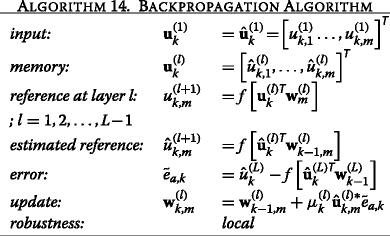But, also the search for worst-case sequences in asymmetric algorithms that exhibit singular values larger than one, can remain inconclusive. Once such a sequence is found, non-robustness follows, but if the search space is too large and no sequence is found in a random or somehow sophisticated search, it remains unclear whether such sequence does not exist or if we simply cannot find it.

Once we need to rely on the algorithms, we should thus turn to the few robust algorithms rather than pray for stability in the mean-square sense. An open question in this contest remains, however:

Is the MSE really the trouble maker, or is it the independence assumption?

As the mean-square analysis typically comes with the independent assumption, both are not easy to separate. The few known cases for which an analysis is known without the independence assumption [71, 72] are valid for the LMS algorithm only (either for very short or infinitely long filters) and this is as we know a very robust algorithm of symmetrical form.

There are indeed many more algorithms that are worth mentioning. They cannot all be named due to limited space. Let us, however, shortly refer to the notion of stable in probability (almost sure convergence), as it provides another means of describing stable or unstable filter behavior. In , the LMS algorithm was analyzed in terms of almost sure convergence, showing that substantially larger step-sizes can be employed than those obtained from MSE analysis. Such methods were successfully applied to the constant modulus algorithm (CMA)  and the least mean fourth (LMF) algorithm , showing that indeed divergence of the algorithms can be readily obtained when modifying the signal properties of noise  and input [77, 78], respectively. Extensions to higher than four exponents can be found in .

## 9 Endnotes

1 It is worth mentioning in a historical context that there were earlier algorithmic proposals by Robbins-Monro  and Kiefer-Wolfowitz  in the direction of stochastic gradient algorithms.

2 For a complex-valued x k , some alternative definition is required.

## References

1. Z Wang, AC Bovik, Mean squared error: love it or leave it? A new look at signal fidelity measures. IEEE Signal Proc. Mag.26(1), 98–117 (2009).

2. G Ungerboeck, Theory on the speed of convergence in adaptive equalizers for digital communication. IBM J. Res. Develop. 16(6), 546–555 (1972).

3. B Widrow, ME Hoff Jr, Adaptive switching circuits. IRE WESCON Conv. Rec.4:, 96–104 (1960).

4. JE Mazo, On the independence theory of equalizer convergence. Bell Syst. Tech. Journal. 58:, 963–993 (1979).

5. E Hänsler, G Schmidt, Acoustic Echo and Noise Control (Wiley, Hoboken, NJ, USA, 2004).

6. R Nitzberg, Application of the normalized LMS algorithm to MSLC. IEEE Trans. Aerosp. Electron. Syst. 21(1), 79–91 (1985).

7. PL Feintuch, An adaptive recursiv LMS filter. Proc. IEEE. 64(11), 1622–1624 (1976).

8. RCJ Johnson, MG Larimore, Comments on and additions to an adaptive recursive LMS filter. Proc. IEEE. 65(9), 1401–1402 (1977).

9. B Widrow, JM McCool, Comments on an adaptive recursive LMS filter. Proc. IEEE. 65(9), 1402–1404 (1977).

10. CJ Johnson, Inf. Theory, IEEE Transac. 25(6), 745–749 (1979). doi:10.1109/TIT.1979.1056097.

11. JJ Shynk, Adaptive IIR filtering. IEEE ASSP Mag. 6(2), 4–21 (1989). doi:10.1109/53.29644.

12. P Kabal, The stability of adaptive minimum mean square error equalizers using delayed adjustment. IEEE Trans. Commun. 31(3), 430–432 (1983).

13. G Long, F Ling, JG Proakis, The LMS algorithm with delayed coefficient adaptation. IEEE Trans. Acoust. Speech Signal Process. 37(9), 1397–1405 (1989).

14. M Rupp, R Frenzel, Analysis of LMS and NLMS algorithms with delayed coefficient update under the presence of spherically invariant processes. IEEE Trans. Signal Process. 42(3), 668–672 (1994). doi:10.1109/78.277860.

15. B Widrow, D Shur, S Shaffer, in Record of the Fifteenth Asilomar Conference on Circuits, Systems and Computers. On adaptive inverse control, (1981), pp. 185–189.

16. M Rupp, AH Sayed, Robust FxLMS algorithm with improved convergence performance. IEEE Trans. Speech and Audio Process. 6(1), 78–85 (1998). doi:10.1109/89.650314.

17. K Tammi, Active control of rotor vibrations by two feedforward control algorithms. J. Dyn. Syst. Meas. Control. 131:, 1–10 (2009).

18. AJ Hillis, Multi-input multi-output control of an automotive active engine mounting system. Proc. Inst. Mech. Eng. Part D: J. Automob. Eng. 225:, 1492–1504 (2011).

19. F Hausberg, S Vollmann, P Pfeffer, S Hecker, M Plöchl, T Kolkhorst, in 42nd International Congress and Exposition on Noise Control Engineering (Internoise 2013). Improving the convergence behavior of active engine mounts in vehicles with cylinder-on-demand engines (Innsbruck, Austria, 2013).

20. F Hausberg, C Scheiblegger, P Pfeffer, M Plöchl, S Hecker, M Rupp, Experimental and analytical study of secondary path variations in active engine mounts. J Sound Vib. 340:, 22–38 (2015).

21. M Rupp, F Hausberg, in Signal Processing Conference (EUSIPCO), 2014 Proceedings of the 22nd European. LMS algorithmic variants in active noise and vibration control, (2014), pp. 691–695.

22. NJ Bershad, Analysis of the normalized LMS algorithm with Gaussian inputs. IEEE Trans. Acoust. Speech Signal Process. 34(4), 793–806 (1986).

23. M Tarrab, A Feuer, Convergence and performance analysis of the normalized LMS algorithm with uncorrelated Gaussian data. IEEE Trans. Inform. Theory. 34(4), 680–691 (1988).

24. M Rupp, The behavior of LMS and NLMS algorithms in the presence of spherically invariant processes. IEEE Trans. Signal Process. 41(3), 1149–1160 (1993). doi:10.1109/78.205720.

25. AH Sayed, Fundamentals of Adaptive Filtering (Wiley, Hoboken, NJ, USA, 2003).

26. M Rupp, Asymptotic equivalent analysis of the LMS algorithm under linearly filtered processes. EURASIP J. Adv Signal Process (2015).

27. HK Khalil, Nonlinear Systems (Mac Millan, US, 1992).

28. M Vidyasagar, Nonlinear Systems Analysis (Prentice Hall, second edition, New Jersey, 1993).

29. B Hassibi, AH Sayed, T Kailath, in Proc. Conference on Decision and Control, 1. LMS is H optimal (San Antonio, TX, 1993), pp. 74–79.

30. AH Sayed, M Rupp, in Proc. SPIE Conf. Adv. Signal Process, 2563. A time-domain feedback analysis of adaptive gradient algorithms via the small gain theorem (San Diego, CA, USA, 1995), pp. 458–469. doi:10.1117/12.211422.

31. M Rupp, AH Sayed, A time-domain feedback analysis of filtered-error adaptive gradient algorithms. IEEE Trans. Signal Process. 44(6), 1428–1439 (1996). doi:10.1109/78.506609.

32. AH Sayed, M Rupp, Error-energy bounds for adaptive gradient algorithms. IEEE Trans. Signal Process. 44(8), 1982–1989 (1996). doi:10.1109/78.533719.

33. AH Sayed, M Rupp, in The Digital Signal Processing Handbook. Robustness issues in adaptive filtering (CRC PressBoca Raton, FL, USA, 1998). Chap. 20.

34. B Hassibi, T Kailath, in Decision and Control, 1994., Proceedings of the 33rd IEEE Conference On, 4. H bounds for the recursive-least-squares algorithm, (1994), pp. 3927–39284. doi:10.1109/CDC.1994.411555.

35. M Rupp, AH Sayed, Robustness of Gauss-Newton recursive methods: a deterministic feedback analysis. Signal Process. 50:, 165–187 (1996). doi:10.1016/0165-1684(96)00022-9.

36. B Hassibi, T Kailath, h bounds for LS estimators. IEEE Trans. Autom. Control. 46:, 309–414 (2001).

37. M Rupp, AH Sayed, Supervised learning of perceptron and output feedback dynamic networks: A feedback analysis via the small gain theorem. IEEE Trans. Neural Netw. 8(3), 612–622 (1997). doi:10.1109/72.572100.

38. K Ozeki, T Umeda, An adaptive filtering algorithm using orthogonal projection to an affine subspace and its properties. Electron. Commun. Japan. 67-A(5), 19–27 (1984).

39. SL Gay, in Third International Workshop on Acoustic Echo Control. A fast converging, low complexity adaptive filtering algorithm (Plestin les Greves, France, 1993).

40. SL Gay, S Tavathia, in Proc. Intl. Conf on Acoustics, Speech and Signal Proc.The fast affine projection algorithm (Detroit, MI, 1995).

41. A Mader, H Puder, G Schmidt, Step-size control for acoustic echo cancellation filters—an overview. Signal Process. 80(9), 1697–1719 (2000).

42. M Rupp, Pseudo affine projection algorithms revisited: robustness and stability analysis. IEEE Trans. Signal Process. 59(5), 2017–2023 (2011). doi:10.1109/TSP.2011.2113346.

43. RW Lucky, Automatic equalization for digital communication. Bell System Technical J. 44:, 547–588 (1965).

44. J Proakis, Digital Communications (McGraw-Hill, New York, 2000).

45. M Rupp, Convergence properties of adaptive equalizer algorithms. IEEE Trans. Signal Process. 59(6), 2562–2574 (2011). doi:10.1109/TSP.2011.2121905.

46. M Rupp, Robust design of adaptive equalizers. IEEE Trans. Signal Process. 60(4), 1612–1626 (2012). doi:10.1109/TSP.2011.2180717.

47. J Benesty, T Gänsler, Y Huang, M Rupp, in Audio Signal Processing for Next-Generation Multimedia Communication Systems, ed. by Y Huang, J Benesty. Adaptive Algorithms for Mimo Acoustic Echo Cancellation (Springer, 2004), pp. 119–147. ISBN: 978-1-4020-7768-5.

48. L Tong, G Xu, T Kailath, in Conference Record of the Twenty-Fifth Asilomar Conference on Signals, Systems and Computers. A new approach to blind identification and equalization of multipath channels, (1991), pp. 856–8602. doi:10.1109/ACSSC.1991.186568.

49. L Tong, S Perreau, Multichannel blind identification: from subspace to maximum likelihood methods. Proc. IEEE. 86(10), 1951–1968 (1998). doi:10.1109/5.720247.

50. Y Huang, J Benesty, Adaptive multi-channel least mean square and Newton algorithms for blind channel identification. Signal Process. 82:, 1127–1138 (2002).

51. M Rupp, AH Sayed, On the convergence of blind adaptive equalizers for constant modulus signals. IEEE Trans. Commun. 48(5), 795–803 (2000). doi:10.1109/26.843192.

52. R Dallinger, M Rupp, in Record 43rd ACSSC. A strict stability limit for adaptive gradient type algorithms (Pacific Grove, CA, USA, 2009), pp. 1370–1374. doi:10.1109/ACSSC.2009.5469884.

53. DL Duttweiler, Proportionate normalized least mean square adaptation in echo cancellers. IEEE Trans. Speech Audio Process. 8(5), 508–518 (2000).

54. S Makino, Y Kaneda, N Koizumi, Exponentially weighted step-size NLMS adaptive filter based on the statistics of a room impulse response. IEEE Trans. Speech and Audio Process. 1(1), 101–108 (1993). doi:10.1109/89.221372.

55. J Benesty, SL Gay, in Proc. IEEE ICASSP. An improved PNLMS algorithm, (2002), pp. 1881–1884.

56. M Rupp, J Cezanne, Robustness conditions of the LMS algorithm with time-variant matrix step-size. Signal Process. 80(9), 1787–1794 (2000). doi:10.1016/S0165-1684(00)00088-8.

57. R Dallinger, M Rupp, in Proc. of the 38th International Conference on Acoustics, Speech, and Signal Processing (ICASSP’13). On the robustness of LMS algorithms with time-variant diagonal matrix step-size, (2013). doi:10.1109/ICASSP.2013.6638754.

58. J Arenas-García, AR Figueiras-Vidal, AH Sayed, Mean-square performance of a convex combination of two adaptive filters. IEEE Trans. Signal Process. 54(3), 1078–1090 (2006). doi:10.1109/TSP.2005.863126.

59. RT Flanagan, J-J Werner, Cascade echo canceler arrangement. U.S. Patent 6,009,083 (1999).

60. DY Huang, X Su, A Nallanathan, in Proc. IEEE ICASSP 2005, 3. Characterization of a cascade LMS predictor (Singapore, Singapore, 2005), pp. 173–176. doi:10.1109/ICASSP.2005.1415674.

61. SC Cripps, Advanced Techniques in RF Power Amplifier Design (Artech House, Inc., Boston (MA), USA, 2002).

62. E Aschbacher, M Rupp, in Proc. IEEE SSP 2005. Robustness analysis of a gradient identification method for a nonlinear Wiener system (Bordeaux, France, 2005), pp. 103–108. doi:10.1109/SSP.2005.1628573.

63. R Dallinger, M Rupp, in Proc. IEEE ICASSP 2010. Stability analysis of an adaptive Wiener structure (Dallas, TX, USA, 2010), pp. 3718–3721. doi:10.1109/ICASSP.2010.5495866.

64. R Dallinger, M Rupp, in Proc. of EUSIPCO Conference. Stability of adaptive filters with linearly interfering update errors, (2015).

65. M Rupp, S Schwarz, in 40th International Conference on Acoustics, Speech, and Signal Processing (ICASSP’15). A tensor LMS algorithm, (2015), pp. 3347–3351. doi:10.1109/ICASSP.2015.7178591.

66. M Rupp, S Schwarz, in Proc. of EUSIPCO Conference. Gradient-based approaches to learn tensor products, (2015).

67. DE Rumelhart, GE Hinton, RJ Williams, Learning representations by back-propagating errors. Nature. 323:, 533–536 (1986). doi:10.1038/323533a0.

68. RP Lippmann, An introduction to computing with neural nets. IEEE Trans. Acoust. Speech Signal Process. 4(2), 4–22 (1987). doi:10.1109/MASSP.1987.1165576.

69. R Rojas, Neural Networks (Springer, Berlin, Germany, 1996).

70. B Hassibi, AH Sayed, T Kailath, in Theoretical Advances in Neural Computation and Learning, ed. by V Roychowdhury, K Siu, and A Orlitsky. LMS and backpropagation are minimax filters (Kluwer Academic PublishersNorwell, MA, USA, 1994), pp. 425–447. Chap. 12.

71. SC Douglas, W Pan, Exact expectation analysis of the LMS adaptive filter. IEEE Trans. Signal Process. 43(12), 2863–2871 (1995). doi:10.1109/78.476430.

72. H-J Butterweck, in International Conference on Acoustics, Speech, and Signal Processing (ICASSP-95), 2. A steady-state analysis of the LMS adaptive algorithm without use of the independence assumption, (1995), pp. 1404–1407. doi:10.1109/ICASSP.1995.480504.

73. VH Nascimento, AH Sayed, in Signals, Systems & Computers, 1998. Conference Record of the Thirty-Second Asilomar Conference on, vol. 2. Are ensemble-average learning curves reliable in evaluating the performance of adaptive filters? (1998), pp. 1171–1175. doi:10.1109/ACSSC.1998.751511.

74. DN Godard, Self-recovering equalization and carrier tracking in twodimensional data communication systems. IEEE Trans. Commun. 28(11), 1867–1875 (1980). doi:10.1109/TCOM.1980.1094608.

75. E Walach, B Widrow, The least mean fourth (LMF) adaptive algorithm and its family. IEEE Trans. Inf. Theory. 30(2), 275–283 (1984). doi:10.1109/TIT.1984.1056886.

76. O Dabeer, E Masry, Convergence analysis of the constant modulus algorithm. IEEE Trans. Inf. Theory. 49(6), 1447–1464 (2003). doi:10.1109/TIT.2003.811903.

77. VH Nascimento, JCM Bermudez, Probability of divergence for the least-mean fourth algorithm. IEEE Trans. Signal Process. 54(4), 1376–1385 (2006). doi:10.1109/TSP.2006.870546.

78. PI Hubscher, JCM Bermudez, VH Nascimento, A mean-square stability analysis of the least mean fourth adaptive algorithm. IEEE Trans. Signal Process. 55(8), 4018–4028 (2007). doi:10.1109/TSP.2007.894423.

79. M Moinuddin, UM Al-Saggaf, A Ahmed, Family of state space least mean power of two-based algorithms. EURASIP J Adv. Signal Process. 39: (2015). doi:10.1186/s13634-015-0219-9.

80. H Robbins, S Monro, A stochastic approximation method. Ann. Math. Stat. 22(3), 400–407 (1951).

81. J Kiefer, J Wolfowitz, Stochastic estimation of the maximum of a regression function. Ann. Math. Stat. 23(3), 462–466 (1952).

## Author information

Authors

### Corresponding author

Correspondence to Markus Rupp.

### Competing interests

The authors declare that they have no competing interests.

### Authors’ information

Markus Rupp received his Dipl.-Ing. degree in 1988 at the University of Saarbrücken, Germany and his Dr.-Ing. degree in 1993 at the Technische Universität Darmstadt, Germany, where he worked with Eberhardt Hänsler on designing new algorithms for acoustical and electrical echo compensation. From November 1993 until July 1995, he had a postdoctoral position at the University of Santa Barbara, California with Sanjit Mitra where he worked with Ali H. Sayed on a robustness description of adaptive filters with impact on neural networks and active noise control. From October 1995 until August 2001 he was a member of Technical Staff in the Wireless Technology Research Department of Bell-Labs at Crawford Hill, NJ, where he worked on various topics related to adaptive equalization and rapid implementation for IS-136, 802.11 and UMTS. Since October 2001 he is a full professor for Digital Signal Processing in Mobile Communications at the TU Wien in Austria where he founded the Christian-Doppler Laboratory for Design Methodology of Signal Processing Algorithms in 2002 at the Institute of Communications and RF Engineering (now Institue of Telecommunications). He served as Dean from 2005–2007 and from 2016–2017. He was associate editor of IEEE Transactions on Signal Processing from 2002–2005, is currently associate editor of JASP EURASIP Journal of Advances in Signal Processing, JES EURASIP Journal on Embedded Systems. He was elected AdCom member of EURASIP from 2004–2012 and serving as president of EURASIP from 2009–2010. He authored and co-authored more than 500 papers and patents on adaptive filtering, wireless communications, and rapid prototyping, as well as automatic design methods. He is a Fellow of the IEEE.